# DetermTable: Deterministic tables

A DetermTable provides an input view like that of an edit table (see To edit a table), allowing you to specify values or expressions in each cell for all index combinations; however, unlike a table, the evaluation of a determtable conditionally returns only selected values from the table. It is called a determtable because it acts as a deterministic function of one or more discrete-valued variables. You can conceptualize a determtable as a multi-dimensional generalization of a select-case statement found in many programming languages, or as a value that varies with the path down a decision tree.

The following shows the edit view of a determ table, in which you can enter a different miles per gallon for each car type. Car_type has been changed from being an index in previous examples to a decision node here, defined as a Choice, with the Hybrid selected.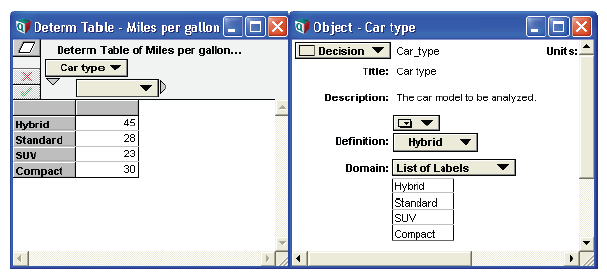When Miles_per_gallon is evaluated, its result contains only the miles per gallon for the selected car type.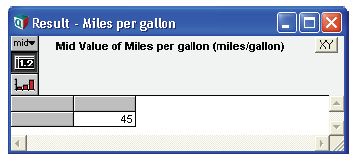In comparison, the result of evaluating a straight table would include all values for all car types.

DetermTable inputs: The dimensions of a determtable may be a combination of normal indexes and discrete variables. Each discrete variable used must have a domain that explicitly contains all possible values, and it is these values that are used for the dimension in the determtable edit view. The selection occurs over the discrete variables, so that DetermTable() behaves differently from Table() only when at least one of the dimensions is a discrete variable. The definition of each discrete variable specifies which value from its domain is selected.

When you define a discrete variable to serve as an input to DetermTable(), it is convenient to use a choice menu (see Creating a choice menu) with the index for the Choice function set to Self. You must then set the domain attribute to either Explicit Values, Copy from index, or Expression. The Explicit Values option allows you to exist all possible values directly. A Copy from index domain pulls the list of possible values from a separate index object that already contains the list of possible values. Expression is used when you want to compute the possible values based on other variables in your model. When using Expression for a DetermTable, the result must be a list or a call to the Discrete() function with the possible values.

Creating a DetermTable: To define a variable as a determtable:

1. Decide on the inputs — the discrete conditioning variables.
2. Press the expr menu above the definition field and select Other....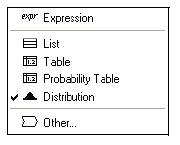Analytica opens the Object Finder dialog.
3. Select Array from the Library popup menu and select Determtable from the function list.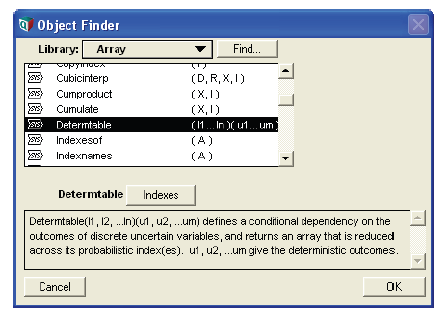4. Click the Indexes button to open the Indexes dialog, which lets you choose discrete conditioning variable(s).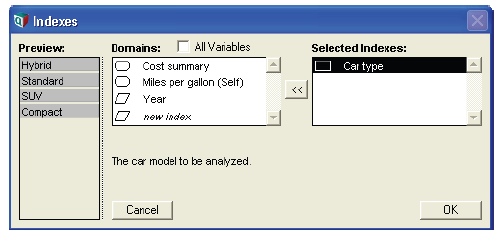5. Click OK to accept the indexes and open an Edit Table window.
6. Enter the outcomes corresponding to each outcome of your discrete inputs.

Expression view of a determtable: When you select the expression view of a definition that was created as a determtable, it looks like this:

Determtable(i1, i2, … in) (r1, r2, r3, … rm)

This describes an n-dimensional conditional deterministic table, indexed by the indexes and discrete conditioning variables «i1», «i2», … «in». The last index, «in», is the innermost index, varying the most rapidly. «r1», «r2», … «rm» are the outcomes in the array.

Converting a Table to a DetermTable: To convert an existing table to a DetermTable, view either the Object window or Attribute panel for the variable and use the pull-down to change the definition type to Other.... Answer Yes when asked to replace the current definition, and the Object Finder dialog appears. From the Array library select DetermTable and press OK.

An alternative way to convert a table to a DetermTable is to view the table definition in expression mode and change the first word Table to DetermTable.

Use in Parametric Analysis: A parametric analysis varies one or more model inputs across several hypothetical values, computing results for each combination of inputs. Array abstraction makes it very easy to conduct parametric analyses in Analytica; however, the computational complexity and memory requirements scale multiplicatively as you vary more and more input variables simultaneously varied.

DetermTables provide a useful tool for coping with the complexity / dimensionality trade-off. You can select a subset of input variables to vary parametrically, examine your model outputs as these vary, then re-run your model after selecting a different subset of inputs to vary. Using Choice menus for the inputs, and DetermTables for any tables based on those input dimensions, makes it possible to change your parametric inputs rapidly to quickly explore relationships elucidated by your model. Obtaining this agility is often a simple matter of converting existing tables to DetermTables.

Subscript equivalence: You can achieve the equivalent functionality of DetermTable without using the DetermTable function, but DetermTable is a nice convenience that saves having an extra node in your model. As an alternative to a DetermTable, you can create a standard Edit table in a variable, A, and then obtain the desired slice in a second variable, B, by defining it as A[u = u, v = v], where u and v are the discrete conditioning variables. This works because u and v are both self-indexed (with the possible values being the self-index values) and also have their own value (the selected value).